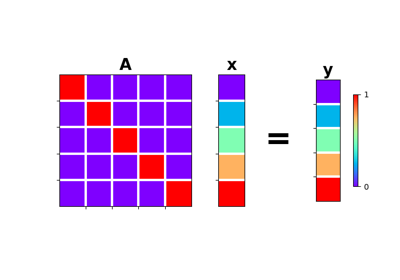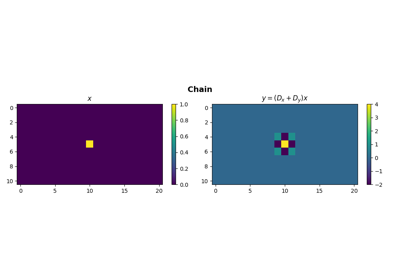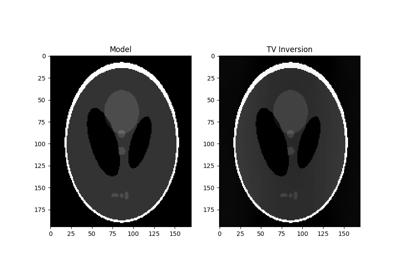# pylops.Identity¶

class pylops.Identity(N, M=None, dtype='float64', inplace=True)[source]

Identity operator.

Simply move model to data in forward model and viceversa in adjoint mode if $$M = N$$. If $$M > N$$ removes last $$M - N$$ elements from model in forward and pads with $$0$$ in adjoint. If $$N > M$$ removes last $$N - M$$ elements from data in adjoint and pads with $$0$$ in forward.

Parameters: N : int Number of samples in data (and model in M is not provided). M : int, optional Number of samples in model. dtype : str, optional Type of elements in input array. inplace : bool, optional Work inplace (True) or make a new copy (False). By default, data is a reference to the model (in forward) and model is a reference to the data (in adjoint).

Notes

For $$M = N$$, an Identity operator simply moves the model $$\mathbf{x}$$ to the data $$\mathbf{y}$$ in forward mode and viceversa in adjoint mode:

$y_i = x_i \quad \forall i=1,2,...,N$

or in matrix form:

$\mathbf{y} = \mathbf{I} \mathbf{x} = \mathbf{x}$

and

$\mathbf{x} = \mathbf{I} \mathbf{y} = \mathbf{y}$

For $$M > N$$, the Identity operator takes the first $$M$$ elements of the model $$\mathbf{x}$$ into the data $$\mathbf{y}$$ in forward mode

$y_i = x_i \quad \forall i=1,2,...,N$

and all the elements of the data $$\mathbf{y}$$ into the first $$M$$ elements of model in adjoint mode (other elements are O):

\begin{align}\begin{aligned}x_i = y_i \quad \forall i=1,2,...,M\\x_i = 0 \quad \forall i=M+1,...,N\end{aligned}\end{align}
Attributes: shape : tuple Operator shape explicit : bool Operator contains a matrix that can be solved explicitly (True) or not (False)

Methods

 __init__(self, N[, M, dtype, inplace]) Initialize this LinearOperator. adjoint(self) Hermitian adjoint. cond(self, \*\*kwargs_eig) Condition number of linear operator. conj(self) Complex conjugate operator div(self, y[, niter]) Solve the linear problem $$\mathbf{y}=\mathbf{A}\mathbf{x}$$. dot(self, x) Matrix-matrix or matrix-vector multiplication. eigs(self[, neigs, symmetric, niter]) Most significant eigenvalues of linear operator. matmat(self, X) Matrix-matrix multiplication. matvec(self, x) Matrix-vector multiplication. rmatvec(self, x) Adjoint matrix-vector multiplication. transpose(self) Transpose this linear operator.

## Examples using pylops.Identity¶IdentityOperators concatenationTotal Variation (TV) Regularization